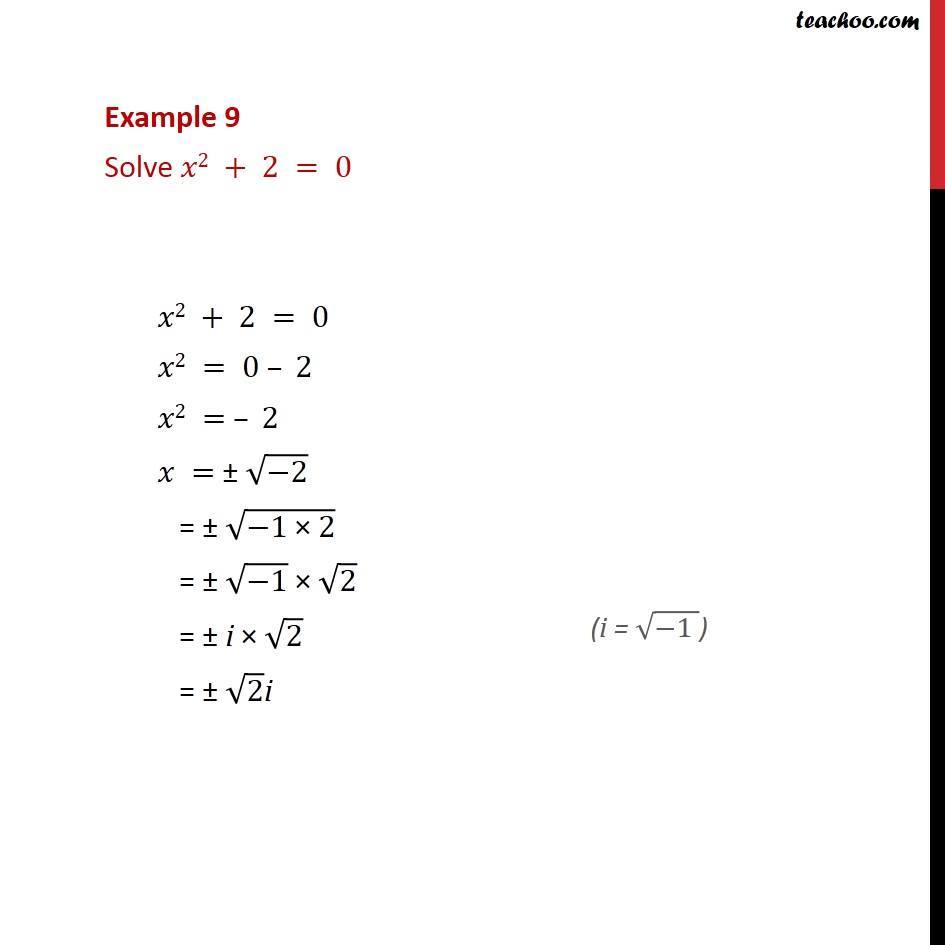Chapter 4 Class 11 Complex Numbers
Concept wiseLearn in your speed, with individual attention - Teachoo Maths 1-on-1 Class

### Transcript

Question 3 - Chapter 5 Class 11 Complex Numbers - NCERT Solve 𝑥^2 + 2 = 0 𝑥2 + 2 = 0 𝑥2 = 0 – 2 𝑥2 = – 2 𝑥 = ± √(−2) = ± √(−1 × 2) (i = √(−1 )) = ± √(−1) × √2 = ± i × √2 = ± √2i